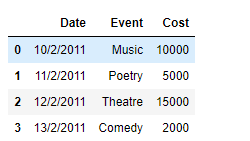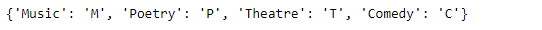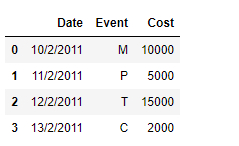Related Articles

# Using dictionary to remap values in Pandas DataFrame columns

• Last Updated : 23 Jan, 2019

While working with data in Pandas, we perform a vast array of operations on the data to get the data in the desired form. One of these operations could be that we want to remap the values of a specific column in the DataFrame. Let’s discuss several ways in which we can do that.

Given a Dataframe containing data about an event, remap the values of a specific column to a new value.

Code #1: We can use `DataFrame.replace()` function to achieve this task. Let’s see how we can do that.

 `# importing pandas as pd``import` `pandas as pd`` ` `# Creating the DataFrame``df ``=` `pd.DataFrame({``'Date'``:[``'10/2/2011'``, ``'11/2/2011'``, ``'12/2/2011'``, ``'13/2/2011'``],``                    ``'Event'``:[``'Music'``, ``'Poetry'``, ``'Theatre'``, ``'Comedy'``],``                    ``'Cost'``:[``10000``, ``5000``, ``15000``, ``2000``]})`` ` `# Print the dataframe``print``(df)`

Output :Now we will remap the values of the ‘Event’ column by their respective codes.

 `# Create a dictionary using which we``# will remap the values``dict` `=` `{``'Music'` `: ``'M'``, ``'Poetry'` `: ``'P'``, ``'Theatre'` `: ``'T'``, ``'Comedy'` `: ``'C'``}`` ` `# Print the dictionary``print``(``dict``)`` ` `# Remap the values of the dataframe``df.replace({``"Event"``: ``dict``})`

Output :Code #2: We can use `map()` function to achieve this task.

 `# importing pandas as pd``import` `pandas as pd`` ` `# Creating the DataFrame``df ``=` `pd.DataFrame({``'Date'``:[``'10/2/2011'``, ``'11/2/2011'``, ``'12/2/2011'``, ``'13/2/2011'``],``                    ``'Event'``:[``'Music'``, ``'Poetry'``, ``'Theatre'``, ``'Comedy'``],``                    ``'Cost'``:[``10000``, ``5000``, ``15000``, ``2000``]})`` ` `# Print the dataframe``print``(df)`

Output :Now we will remap the values of the ‘Event’ column by their respective codes.

 `# Create a dictionary using which we``# will remap the values``dict` `=` `{``'Music'` `: ``'M'``, ``'Poetry'` `: ``'P'``, ``'Theatre'` `: ``'T'``, ``'Comedy'` `: ``'C'``}`` ` `# Print the dictionary``print``(``dict``)`` ` `# Remap the values of the dataframe``df[``'Event'``]``=` `df[``'Event'``].``map``(``dict``)`` ` `# Print the DataFrame after modification``print``(df)`

Output :Attention geek! Strengthen your foundations with the Python Programming Foundation Course and learn the basics.

To begin with, your interview preparations Enhance your Data Structures concepts with the Python DS Course. And to begin with your Machine Learning Journey, join the Machine Learning – Basic Level Course

My Personal Notes arrow_drop_up